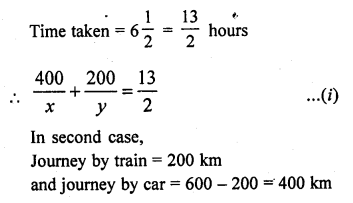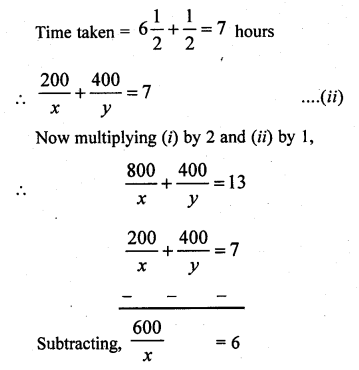• +91 9971497814
• info@interviewmaterial.com

# RD Chapter 3- Pair of Linear Equations in Two Variables Ex-3.10 Interview Questions Answers

### Related Subjects

Question 1 : Points A and B are 70 km. a part on a highway. A car starts from A and another car starts from B simultaneously. If they travel in the same direction, they meet in 7 hours, but if they travel towards each other, they meets in one hour. Find the speed of the two cars. (C.B.S.E. 2002)

Distance between two points A and B = 70 km
Let speed of first car starting from A = x km/hr
and speed of second car starting from B =y km/hr
When these start in the some direction, they meet after 7 hours
Distance travelled by the first car = 7x km
and by second car = 7y km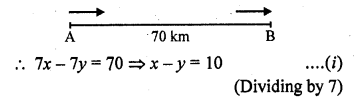When these travel in opposite direction, they meet after 1 hour then distance travelled by first car = x km
and by second car = y km
x + y = 70 ….(ii)
2x = 80
=> x = 40
and subtracting (i) from (ii)
2y = 60
=> y = 30
Speed of first car = 40 km/hr
and speed of second car = 30 km/hr

Question 2 : A sailor goes 8 km downstream in 40 minutes and returns in 1 hour. Determine the speed of the sailor in still water and the speed of the current. (C.B.S.E. 1997)

Let the speed of sailor in still water = x km/ hr
and speed of water = y km/hr
Distance covered = 8 km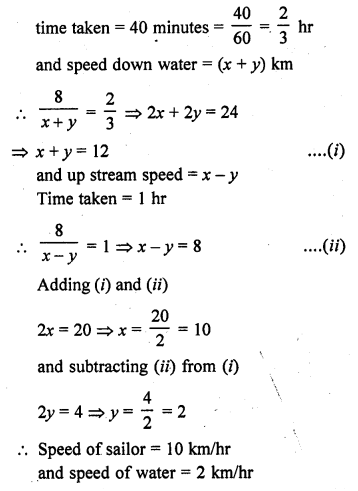Question 3 : The boat goes 30 km upstream and 44 km downstream in 10 hours. In 13 hours, it can go 40 km upstream and 55 km downstream. Determine the speed of stream and that of the boat in still water.

Let the speed of stream = y km/hr
and speed of boat = x km/hr
Speed of boat downstream = (x + y) km/hr
In first case, and up stream = (x – y) km/hr
Upstream distance = 30 km
and down distance = 44 km
Total time taken = 10 hrs
In second case,
upstream distance = 40 km
and downstream distance = 55 km
Total time taken = 13 hrs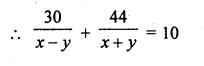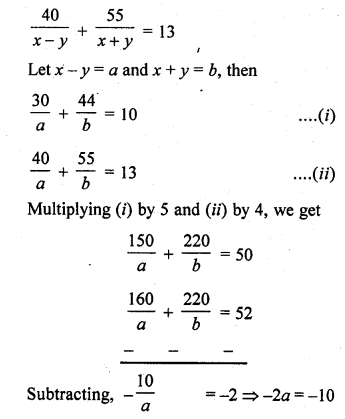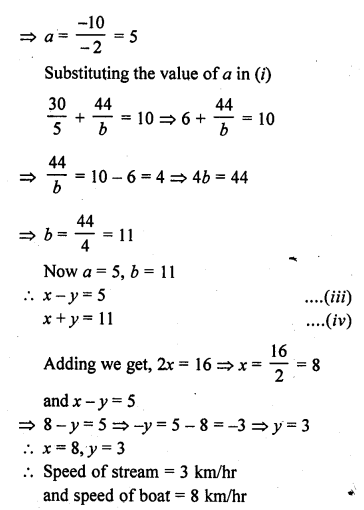Question 4 : A boat goes 24 km upstream and 28 km downstream in 6 hrs. It goes 30 km upstream and 21 km downstream in 6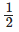hrs. Find the speed of the boat in still water and also speed of the stream.

Let the speed of boat = x km/hr
and speed of stream = y km/hr
In first case,
Distance covered upstream = 24 km
and down stream = 28 km
Total time taken = 6 hours
In second case,
Distance covered upstream = 30 km
and downstream = 21 km
Total time taken = 6= 13/2 hrs.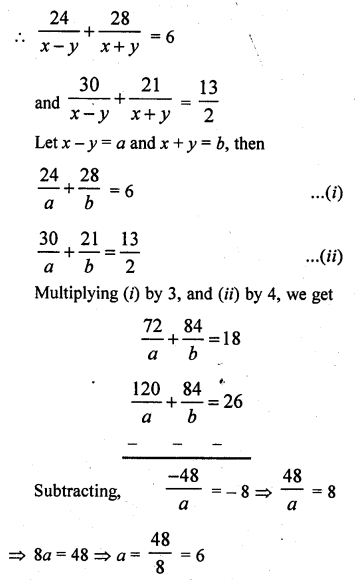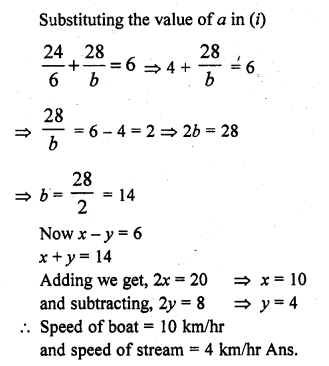Question 5 : A man walks a certain distance with a certain speed. If he walks 1/2 km an hour faster, he takes 1 hour less. But, if he walks 1 km an hour slower, he takes 3 more hours. Find the distance covered by the man and his original rate of walking.

Let the distance = x km
and let certain speed = y km/hr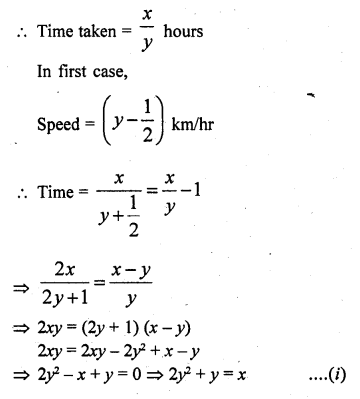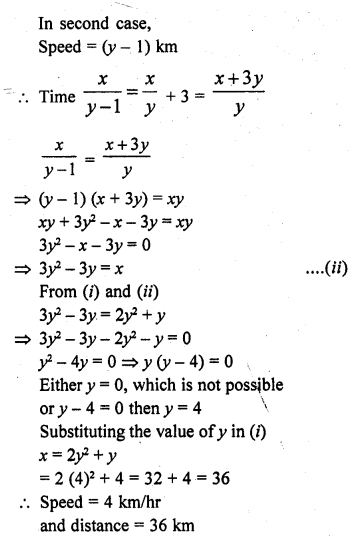Question 6 : A person rowing at the rate of 5 km/h in still water, takes thrice as much time in going 40 km upstream as in going 40 km downstream. Find the speed of the stream. [NCERT Exemplar]

Let the speed of the stream be v km/h
Given that, a person rowing in still water = 5 km/h
The speed of a person rowing in downstream = (5 + v) km/h
and the speed of a person Has rowing in upstream = (5 – v) km/h
Now, the person taken time to cover 40 km downstream,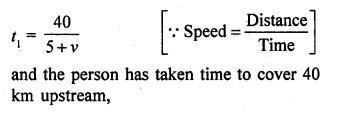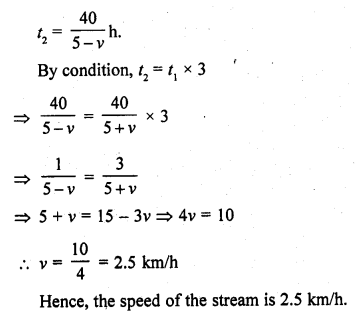Question 7 : Ramesh travels 760 km to his home partly by train and partly by car. He takes 8 hours if he travels 160 km. by train and the rest by car. He takes 12 minutes more if the travels 240 km by train and the rest by car. Find the speed of the train and car respectively.

Total distance = 769 km
Let the speed of train = x km/hr
and speed of car = y km/hr
Time taken = 8 hours
In first case, distance travelled by train = 160 km
and rest distance 760 – 160 = 600 km by car
Time taken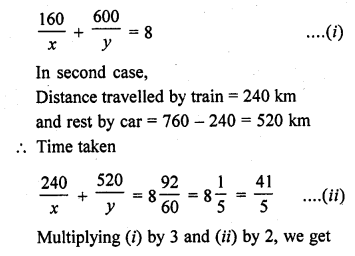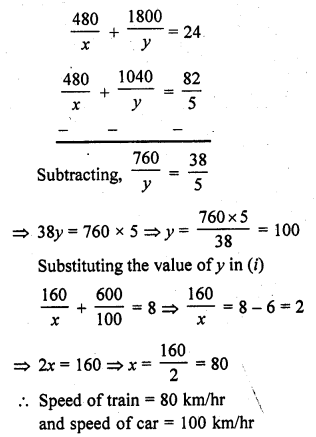Question 8 : A man travels 600 km partly by train and partly by car. If the covers 400 km by train and the rest by car, it takes him 6 hours and 30 minutes. But, if he travels 200 km by train and the rest by car, he takes half an hour longer. Find the speed of the train and that of the car. (C.B.S.E. 2001)